### Showing videos fromEddie Woo with a total of 3,991 videos

12:17Set Theory Diagrams (2 of 2: Venn & Carroll Diagrams)
4y9m ago
04:13Set Theory Diagrams (1 of 2: The Importance of Visual Language)
4y9m ago
13:42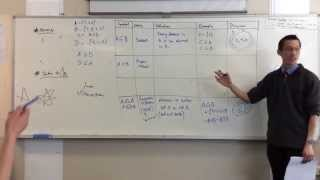Introduction to Set Theory (3 of 3: Further Language & Notation)
4y9m ago
13:31Introduction to Set Theory (2 of 3: Set Operators)
4y9m ago
04:05Introduction to Set Theory (1 of 3: Topic Rationale)
4y9m ago
07:55Finding Angles with Trigonometry (2 of 2: Using the Calculator)
4y9m ago
04:50Finding Angles with Trigonometry (1 of 2: What's an Inverse?)
4y9m ago
03:56Iterated Function Systems (4 of 4: The Mandelbrot Set)
4y9m ago
13:39Iterated Function Systems (3 of 4: Bounded & Unbounded Dynamic Sequences)
4y9m ago
07:03Iterated Function Systems (2 of 4: The Special Case of f(z)=z²+c)
4y9m ago
11:54Iterated Function Systems (1 of 4: Introduction)
4y9m ago
06:24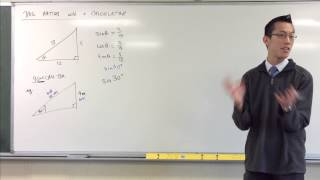Evaluating Trigonometric Ratios with a Calculator
4y9m ago
10:16Using the Trigonometric Ratios (some fundamental examples)
4y9m ago
10:36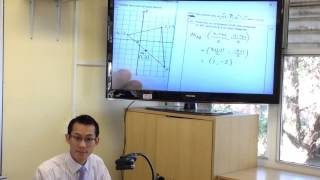Understanding a triangle through Coordinate Geometry
4y9m ago
06:16Plotting points & sketching graphs on the Cartesian plane
4y9m ago
03:51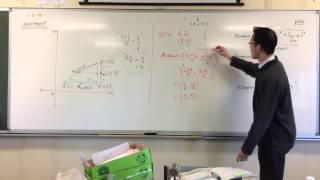Finding the Midpoint (3 of 3: Using the Formula)
4y9m ago
04:28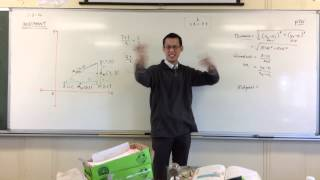Finding the Midpoint (2 of 3: Between 2 Random Points)
4y9m ago
09:29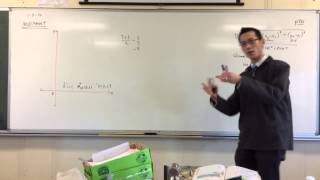Finding the Midpoint (1 of 3: Horizontal & Vertical Intervals)
4y9m ago
07:47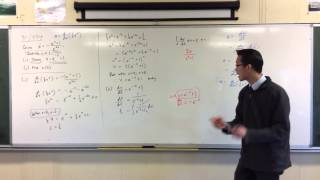Motion Exam Question (2 of 2: Finding x(t) from v(x))
4y9m ago
09:13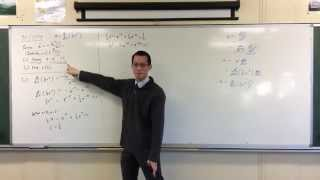Motion Exam Question (1 of 2: Finding v(x) from a(x))
4y9m ago
06:01Calculating Distance (5 of 5: Using the Formula)
4y9m ago
05:08Calculating Distance (4 of 5: Developing the Formula)
4y9m ago
05:04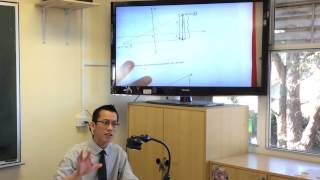Calculating Distance (3 of 5: Example w/ Negative Coordinates)
4y9m ago
05:56Calculating Distance (2 of 5: Using Pythagoras w/ Coordinates)
4y9m ago
06:18Calculating Distance (1 of 5: Review of Pythagoras' Theorem)
4y9m ago
12:56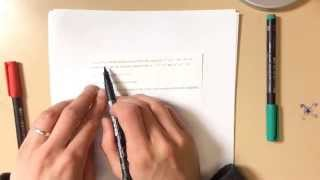Polynomials w/ Complex Roots (interesting exam question)
4y9m ago
04:09Volume of a Tetrahedron (by similar cross-sections)
4y9m ago
05:23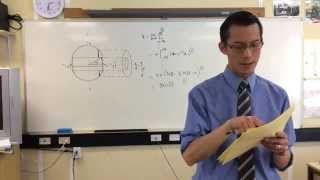Useful Tricks for Evaluating Integrals from a Volume
4y9m ago
04:46Volumes by Slices (example question from exam: hole drilled through sphere)
4y9m ago
06:49Volumes by Cylindrical Shells (example question from exam)
4y9m ago
03:30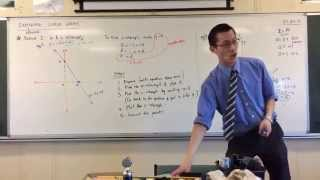Sketching Linear Graphs by x/y-Intercepts Method (2 of 2: Example)
4y9m ago
08:00Sketching Linear Graphs by x/y-Intercepts Method (1 of 2: Explanation)
4y9m ago
05:09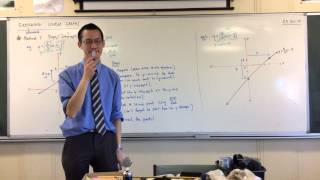Sketching Linear Graphs by Slope/Intercept Method (2 of 2: Example)
4y9m ago
09:27Sketching Linear Graphs by Slope/Intercept Method (1 of 2: Explanation)
4y9m ago
11:16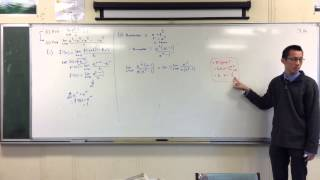Interesting Exponentials Exam Question (2 of 2: Simplifying & Determining the Series Limit)
4y9m ago
03:31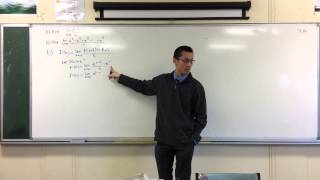Interesting Exponentials Exam Question (1 of 2: Evaluating the Limit)
4y9m ago
04:38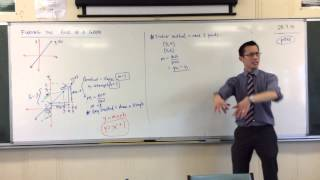Finding the rule (equation) of a graph (2 of 2: Formula Method)
4y9m ago
09:43Finding the rule (equation) of a graph (1 of 2: Triangle Method)
4y9m ago
02:19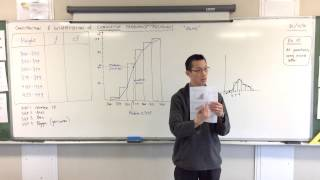Interpreting an Ogive & Building a Cumulative Frequency Table
4y9m ago
11:05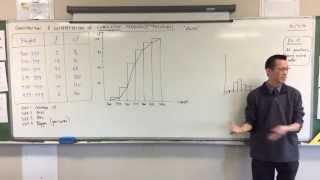Constructing an Ogive (2 of 2: drawing the bars & polygon)
4y9m ago
05:25Constructing an Ogive (1 of 2: setting up data & axes)
4y9m ago